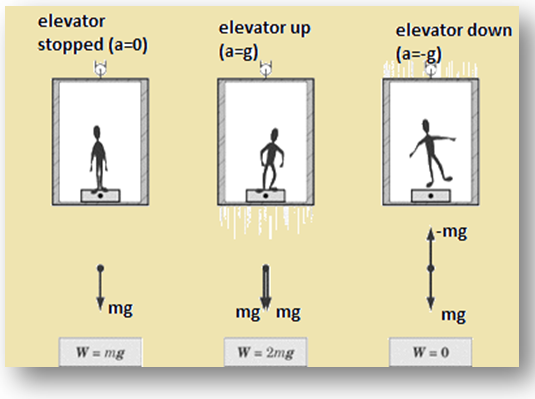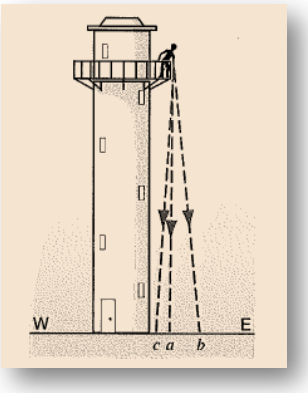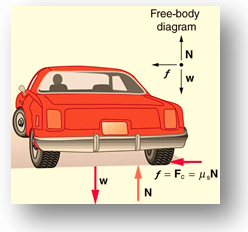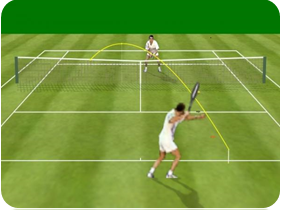Click to Chat

1800-1023-196

+91-120-4616500

CART 0

• 0

MY CART (5)

Use Coupon: CART20 and get 20% off on all online Study Material

ITEM
DETAILS
MRP
DISCOUNT
FINAL PRICE
Total Price: Rs.

There are no items in this cart.
Continue Shopping• Complete Physics Course - Class 11
• OFFERED PRICE: Rs. 2,968
• View Details

Frame of Reference

Table of Content

Inertial and Non-Inertial Frame of Reference

Simulation for Frame of Reference

Conceptual Problem

Related Resources

A system of co-ordinates whose axes can be suitably chosen is said to be a frame of reference. For location of a point ‘P’ we need three co-ordinate x, y and z. For complete identification of an event we must know ‘t’ also, i.e., the time of the occurrence. Hence an event in characterized by four co-ordinates (x,y,z,t). A reference frame describing an event in these four co-ordinates is known a space time frame.Inertial and Non-Inertial Frame of Reference

In general we solve the problem of mechanics using inertial frame, which was discussed in chapter two, but as the same time it is possible to solve the same problem using a non-inertial frame. Let us discuss about the difference between these frames.

When Newton stated his first law he made a very important distinction. He decreed the absolute equivalence between a state of rest and one f uniform motion and distinguished it specifically and absolutely from that of an accelerated motion. If the environment is completely symmetric then no direction is preferred over another and therefore if a body possesses a initial velocity (which might be zero) it will persist with that velocity. If suppose we say that the velocity will change then we will have to concede that the velocity changes in a particular direction. But why should it change in one direction and not in the other, since all direction are equally favored. So the only way it can change is to change in all directions. But this is impossible so it will not change at all, i.e. if environment is really symmetric. Therefore if we grant a change in velocity we will also have to grant an irregularity in the environment in the same direction as the change in velocity. The acceleration, he said to be understood as an irregularity and he expressed force as that basic asymmetry in the environment which produces this irregularity. The most important aspect in all this is that force is theoretical construction to explain away the irregularities in motion and is not to be understood as a tangible entity.

Now, for constant mass system

If force is a tangible entity then the force in all systems on the same body should be same. Let us see if this is true.

Consider a body of mass m. We will observe its motion from three different frames.

Simulation for Frame of Reference

(i)  Reference frame is at rest:-

The acceleration of the mass will be, say, .

Therefore the force on it will be .

We will reason that(ii) Reference frame starts moving with constant velocity vector-v :-

The acceleration of frame =

Thus, acceleration of mass m relative to frame is given by

Force on it will be inertial  and we will reason that

(iii) Reference frame moves with constant acceleration:-

Let the acceleration of frame be  .

Thus, acceleration of mass relative to frame will be .

Let there be force  frame on mass we will reason, that

We see that the force is not the same as that in the inertial frames.

Therefore we postulate that under observation from an accelerated reference frame we substitute the inertial forces on the body with those same initial forces plus an additional force which numerically equal to the mass of the body under observation times the acceleration of the frame taken in the opposite direction. This force we call as pseudo force.

Now, we can work on a problem from an accelerated reference frame by just adding a pseudo force and pretending that nothing has changed.

Let us illustrate our point.

(a) Inertial Frame:-

A frame of reference either at rest or moving with a uniform velocity (zero acceleration) is known as inertial frame. All the laws of physics hold good in such a frame.(b) Non-Inertial or Accelerated Frame:-

It is a frame of reference which is either having a uniform linear acceleration or is being rotated with uniform speed.An inertial frame is endowed with the following characteristics:

All the fundamental laws of physics are valid in inertial frames.
All the fundamental laws of physics assume the same mathematical shape in all inertial frames.
Inertial frames are isotopic with respect to mechanical and optical experiments
The optical experiments performed in an inertial frame in any direction will always yield the same results.

Is earth an inertial frame of reference?Earth rotates around its axis as also revolves around the sun. In both these motion, centripetal acceleration is present. Therefore, strictly speaking earth or any frame of reference fixed on earth cannot be taken as an inertial fame. However, as we are dealing with speeds  ≈ x 108 ms-1 (speed of light) and speed of earth is only about 3 x 104 m/s, therefore when small time intervals are involved effect of rotation and revolution of earth can be ignored. Furthermore, this speed of earth can be assumed to be constant. Hence earth or any other frame of reference set up on earth can be taken as an approximately inertial frame of reference.

On the contrary, a frame of reference which is accelerated or decelerated is a non-inertial frame.

Other examples of inertial frames of reference are:

(i)     A frame of reference remaining fixed w.r.t. stars.

(ii)    A space ship moving in outer space, without spinning and with its engine cut off.

Apparent weight of a man inside a lift:-(a) The lift possesses zero acceleration (fig-1): W = mg

(b) The lift moving upward with an acceleration a (fig-2):

W = mg + ma

= mg + mg

= 2 mg

(c) The lift moving downward with an acceleration a (fig-3):

W = mg – ma

= mg – mg

= 0

Conceptual Problem

Problem 1:-

Suppose that you are standing on the balcony of a tall tower, facing east. You drop an object so that it falls to the ground below; see below figure. Suppose also that you can locate the impact point very precisely. Will the object strike the ground at a, vertically below the release point, at b to the east, or at c to the west? The object was released from rest; the Earth rotates from west to east.Solution:-

If one assumes that the factor by which the Earth rotates is negligible during the time the object takes to reach the ground, then the object will hit the ground at point a and one will not have to concern about the Carioles Effect.

In case the factor is non-negligible, and the Earth moves from West to East, the object will hit the ground at point c. The situation is similar to the perceived leftward displacement of the air moving to a low pressure point from north to south. To the person standing on the balcony of a tall tower, a psuedo force has acted on the object in a direction from east to west.

____________________________________________________________________________________________________

Problem 2:-

What is the distinction between inertial reference frames and those differing only by a translation or rotation of the axes?

Solution:-

One can distinguish the inertial frame of reference against the translation of the axes when the translation or rotation occurs non-uniformly.

If the translation of one frame of reference relative to the other is such that observer in one frame measures some acceleration of the other, then the observer can draw a distinction between his frame and the inertial frame of reference.

It is important to note that the rotating frame will always be non-inertial because to account for the rotation there must be a change in velocity vector. Therefore the inertial reference frame will always be distinct from the rotation frame.

However the translation can be distinguished from the inertial frame of reference only when the translation occurs at a uniform velocity.

_____________________________________________________________________________________________________

Problem 3:-

A passenger in the front seat of a car finds himself sliding toward the door as the driver makes a sudden left turn. Describe the forces on the passenger and on the car at this instant if the motion is viewed from a reference frame (a) attached to the Earth and (b) attached to the car.

Solution:-

(a) From the reference frame attached to the Earth, when the car takes a left turn, the inertia of the passenger would maintain the state of motion of the passenger (in accordance with Newton’s first law of motion). This causes the passenger to move in the direction of velocity vector of car before car took a turn.

Therefore, to the observer at Earth frame, the passenger has the initial velocity equal to the velocity of the car before it takes the turn. The observer also see the car taking a turn, and accounts for an acceleration for the same. Thus, the observer would expect frictional forces to exist between the passenger and the seat.Given that the passenger has moved towards the door when the car took a left turn, it is obvious that the passenger moves to the right. Therefore the frictional force would be in a direction opposite to the motion of the passenger, that is, towards the left.

If you assume that the frictional force between the passenger and seat is given by f , mass of passenger by m whereas the acceleration by a. Then the equation depicting the motion of passenger relative to the observer in Earth’s frame is:

f = ma

Therefore the magnitude of frictional force between the passenger and the seat is equal to the magnitude of the deceleration of the passenger.

(b) According to the observer in car’s frame, the car experiences a centripetal acceleration when it turns to left. Therefore a psuedo force will act on the passenger, accelerating him in the direction of the seat. But there is a friction between the seat of the car and the passenger, which decelerate the passenger in a direction opposite to the direction of its motion.

Thus the magnitude of net force acting on the passenger is the difference between magnitude the centrifugal force and magnitude the frictional force.

If you assume that the centrifugal force acting on passenger is F, the frictional force between the passenger and the seat is f , the mass of passenger is m whereas the acceleration is a. Then the equation depicting the motion of passenger relative to the observer in car’s frame is:

F – f = ma

Therefore one can see that the perceived acceleration for the observer in car differs from that of the observer on Earth.

__________________________________________________________________________________________________

Problem 4:-

Do you have to be concerned with the carioles  effect when playing tennis or golf? If not, why not?Solution:-

No, one does not have to concern with the Carioles Effect when playing tennis or golf because the factor by which the Earth rotates during the time the ball goes from its source to the destination, is very small and can be neglected. Therefore the player can take his/her shot as if the destination of the ball would be at the same position as it was at the time the shot was taken.Question 1:-

A reference frame attached to the earth:

(a) is an inertial frame by definition

(b) cannot be an inertial frame because earth is revolving round the sun

(c) is an inertial frame because Newton’s law are applicable

(d) cannot be an inertial frame because the earth is rotating about its own axis.Question 2:-

Which of the following will not depend on orientation of frame of reference:

(a) a scalar

(b) a vector

(c) the magnitude of a vector

(d) component of a vector

Question 3:-

A vector does not change if :

(a) frame of reference is translated

(b) frame of reference is rotated

(c) vector is translated parallel to itself

(d) vector is rotated through an angle other than multiple of 2π.

Question 4:-

A boy sitting in a moving train throws a ball straight up into the air. The ball falls behind him. So the train is

(a) ascending a hill at constant velocity

(b) descending a hill at constant velocity

(c) having accelerated motion

(d) having retarded motionQ.1
Q.2
Q.3
Q.4

b,d

a,b,c

a,b,c

c

Related Resources

You might like to refer Momentum.

For getting an idea of the type of questions asked, refer the  Previous Year Question Papers.

To read more, Buy study material of Laws of Motion comprising study notes, revision notes, video lectures, previous year solved questions etc. Also browse for more study materials on Physics here.### Course Features

• 101 Video Lectures
• Revision Notes
• Previous Year Papers
• Mind Map
• Study Planner
• NCERT Solutions
• Discussion Forum
• Test paper with Video Solution